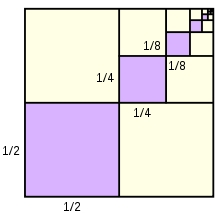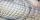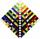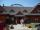# Finite arithmetic sequence

How many numbers should be inserted between the numbers 1 and 25 so that all numbers create a finite arithmetic sequence and that the sum of all members of this group is 117?

n =  7

### Step-by-step explanation:Did you find an error or inaccuracy? Feel free to write us. Thank you!Tips to related online calculators
Do you have a linear equation or system of equations and looking for its solution? Or do you have a quadratic equation?

## Related math problems and questions:

• Sum of the seventeen numbersThe sum of the 17 different natural numbers is 154. Determine the sum of the two largest ones.
• The sumThe sum of the first 10 members of the arithmetic sequence is 120. What will be the sum if the difference is reduced by 2?
• StatisticsThe sum of all deviations from the arithmetic mean of the numerical sequence 4, 6, 51, 77, 90, 93, 95, 109, 113, 117 is:
• Geometric progressionIn geometric progression, a1 = 7, q = 5. Find the condition for n to sum first n members is sn≤217.
• Find the 21Find the sum of the six terms of the finite geometric sequence 96, -48, 24, -12
• SequenceBetween numbers 1 and 53 insert n members of the arithmetic sequence that its sum is 702.
• Geometric sequence 4It is given geometric sequence a3 = 7 and a12 = 3. Calculate s23 (= sum of the first 23 members of the sequence).
• Three members GPThe sum of three numbers in GP (geometric progression) is 21 and the sum of their squares is 189. Find the numbers.
• NumbersDetermine the number of all positive integers less than 4183444 if each is divisible by 29, 7, 17. What is its sum?
• Find the sumFind the sum of all natural numbers from 1 and 100, which are divisible by 2 or 5
• Manufacturing companyA random sample of 8 manufacturing companies is selected from a population of manufacturing companies. The market values (in millions of rands) of these 8 manufacturing companies are: 17 65 117 206 172 181 221 94 What is the lowest and highest market valu
• Sum of membersWhat is the sum of the first two members of the aritmetic progression if d = -4.3 and a3 = 7.5?
• SeriesYour task is express the sum of the following arithmetic series for n = 14: S(n) = 11 + 13 + 15 + 17 + ... + 2n+9 + 2n+11
• AS sequenceIn an arithmetic sequence is given the difference d = -3 and a71 = 455. a) Determine the value of a62 b) Determine the sum of 71 members.
• MotelThree different dinners A, B, C are served in the motel. Three 20 members went to motel. Group 1 ordered dinners: A 20 members and their average dinner price in the group was CZK 200. From Group 2, they ordered a A: 10 persons, B 10 dinners, and their ave
• Sequence 3Write the first 5 members of an arithmetic sequence: a4=-35, a11=-105.
• SequenceIn the arithmetic sequence is given: Sn=2304, d=2, an=95 Calculate a1 and n.